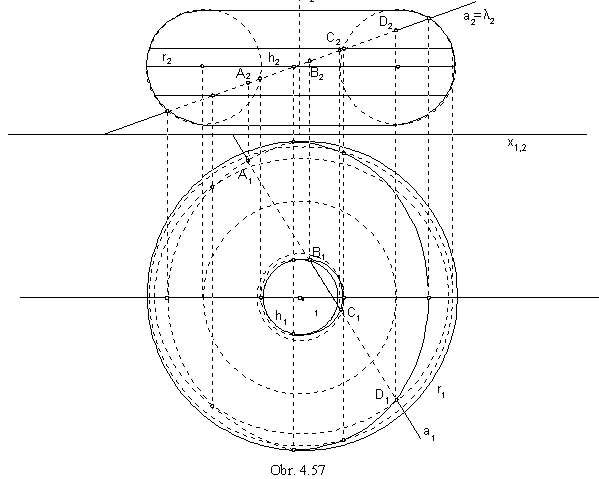Special types of Surfaces of Revolution

Revolving the line a about the axis o there can be created:

cylindrical surface of revolution for a || o

conical surface of revolution for a ´ o

one-sheet hyperboloid of revolution for a / o.

Revolving the circle k(S, r) about the axis o there can be created:

sphere for S Î o

torus for o and k located in one plane, S not located on o.

Revolving the conic section about one of its axes the quadratic surface of revolution can be created.

.1. Cylindrical surface of revolution

Revolving the line segment AB about the parallel axis o there can be created the patch of the cylindrical surface of revolution (Fig. 4. 51).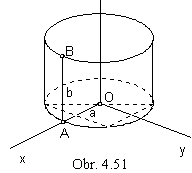Synthetic representation: ( AB, Toz(v) )

Analytic representations:

basic figure - A=(a, 0, 0, 1), B=(a, 0, b, 1)

r(u)=(a, 0, bu,1), uÎ<0,1>

generating principle - class of revolutions about the coordinate axis z

TOz(v), vÎ<0,1>,(0, 2p>

modelled figure - p(u, v) = r(u).TOz(v)=(acos av, asin av, bu, 1), (u,v)Î<0,1>2

2. Conical surface of revolution

Revolving the line segment AB about the intersecting axis o there can be created the patch of the conical surface of revolution (Fig. 4. 52).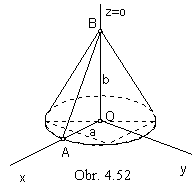Synthetic representation: ( AB, Toz(v) )

Analytic representations:

basic figure - A=(a, 0, 0, 1), B=(0, 0, b, 1)

r(u)=(a(1- u), 0, bu, 1), uÎ<0,1>

generating principle - class of revolutions about the coordinate axis z

TOz(v), v Î<0,1>,<0, 2p>

modelled figure - p(u, v) = r(u).TOz(v) = (a(1-u)cos av, a(1-u)sin av, bu, 1), (u,v)Î<0,1>2

3. One-sheet hyperboloid of revolution

Revolving the line segment AB about the skew axis o there can be created the patch of the one-sheet hyperboloid of revolution (Fig. 4. 53).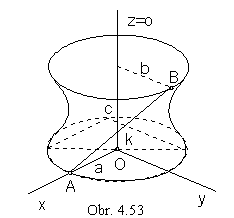Synthetic representation: ( AB, Toz(v) )

Analytic representations:

basic figure - A=(a, 0, 0, 1), B=(0, b, c, 1)

r( u)=(a(1- u), bu, cu,1), u Î<0,1>

generating principle - class of revolutions about the coordinate axis z

TOz(v), vÎ<0,1>,<0, 2p>

modelled figure - p(u, v)= r(u).TOz(v) =

= ( a(1- u)cos av - busin av, a(1- u)sin av + bucos av, cu, 1), (u,v)Î<0,1>2

4. Sphere

Revolving the circular arc k about the axis o located in the plane of the circular arc there can be created a spherical surface patch (Fig. 4. 54).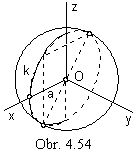Synthetic representation: ( circular arc k(O, a)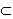n, Toz(v) )

Analytic representations:

basic figure - r(u) = (a cos t, 0, a sin t, 1)

t = -pu +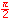for the semicircle in the frontal plane, a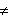0

generating principle - class of revolutions about the coordinate axis z

TOz(v), v Î<0, 1>,<0, 2p>

Modelled figure - p(u, v) = r(u).TOz(v) = (a cos t cos av, a cos t sin av, a sin t, 1)

(u, v)Î<0, 1>2 , a is the radius of the spherical patch

5. Torus

Revolving the circle k about the axis o located in the plane of the basic circle there can be created a torus (Fig. 4. 55).

Synthetic representation: ( k (A, r)n, TOz(v) )

Analytic representations:

basic figure - A=(a, 0, 0, 1), a0, r0

r(u)=(a+r cos 2pu, 0, r sin 2pu, 1)

generating principle - class of revolutions about the coordinate axis z

TOz(v), v Î<0, 1>, aÎ<0, 2p>

modelled figure - p(u, v) = r(u).TOz(v) = ((a+r cos 2pu)cos av, (a+r cos 2pu)sin av, r sin 2pu, 1)

(u, v)Î<0,1>2 , a=2p for the whole torus, r is the radius of the torus

a > r torus - anuloid, a=r axoid, a < r melonoid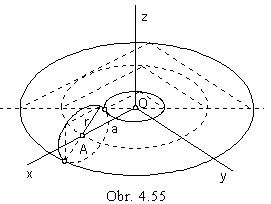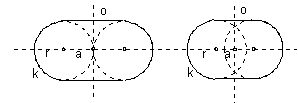Axonometric view of the torus with the axis in the coordinates axis y is in the fig. 4. 56.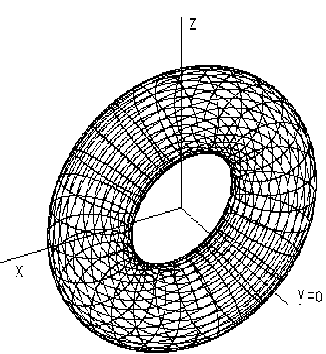Fig. 4. 56

Planar intersection of the torus is the curve (a pair of curves) symmetric with respect to the torus axis called Perseus curve. Points on the intersection curve can be constructed as intersection points of the parallel circles on the torus with the plane of intersection, it means, with the principle lines of the first frame, in which the planes of parallel circles perpendicular to the torus axis intersect the plane of intersection.

Intersection points of the line a and torus with the axis parallel to the coordinate axis z (Fig. 4. 57) can be determined in the frontal projecting plane l located in the line a, which intersects torus in the curve projected to the front view as the line segment. Points on the intersection curve ar points on torus, located on parallel circles, front views of which are line segments perpendicular to the front view of the torus axis, and ground views are circles with the common centre in the ground view of the axis. Intersection points of the line a and torus have their ground views in the intersecion points of the line a ground view and the ground view of the intersection curve. Visibility of the intersection determines the visibility of the line a with respect to the torus. Points of the change of visibility in the ground view are common points of the intersection curve and the neck and equator circles.